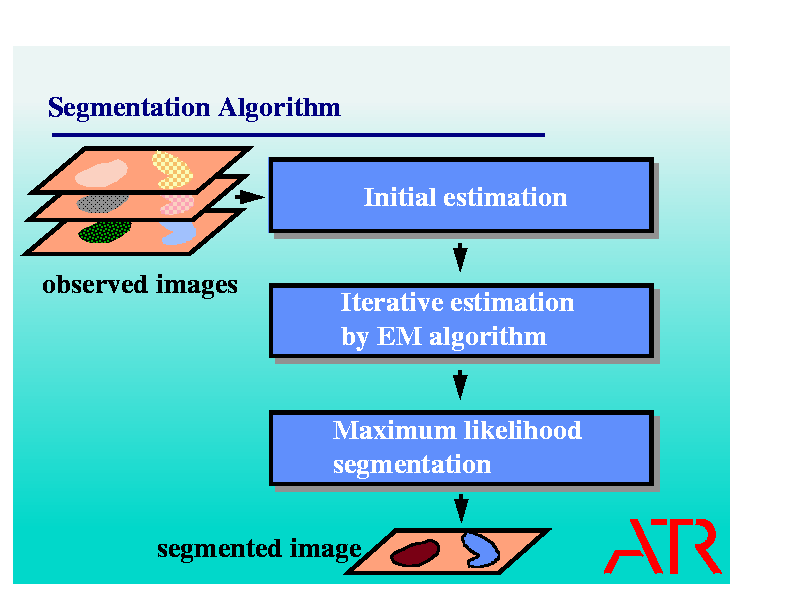Adaptive Color Image Segmentation for Object Coding

Purpose

Hitherto many image compression techniques have been developed and been used to reduce the resource requirements for transmitting data. However mobile communications in wireless environments require much higher compression ratio to save network resources. One possible way to realize the higher compression ratio is to discriminate objects in an image and compress only the necessary objects for users. Scenes change time-dependently and contents of the scene also change. So it is difficult to prepare prior information for the contents and image segmentation methods on the basis of the observed data information is required for discrimination of objects.
When a classification method uses sample image data which are representative of each class, it is called supervised, while a classification method without using sample data is called unsupervised. We shall investigate the unsupervised classification problem, that is also called clustering problem, from the viewpoint of pixel classification base. Here a color image segmentation method is proposed on the basis of the maximum likelihood (ML) estimation for the clustering problem. The difficulty lies in how to estimate the parameters of the likelihood functions and the expectation and maximization (EM) algorithm is introduced to estimate the parameters.

Assumptions

The number of segmentations M to be segmented is assumed to be known. Also, in STEP 2 described in the next paragraph, it is assumed that the observable color image is considered a realization of a mixture of multi-variate normal densities, where the form of a mixture of multi-variate normal density functions is known, but their parameters (mean vectors and covariance matrices) are unknown.

The Segmentation Algorithm

The proposed clustering algorithm is depicted in the figure.
In STEP 1, initial estimates are calculated using a multi-dimensional histogram and the minimum distance clustering method. The multi-dimensional histogram is constructed by dividing the color space into intervals and counting the number of elements of the observed color image in each subspace, which is called a bin. Then the M highest-density bins are selected and the averages of observed elements belonging to the bins are calculated. The average is also called the centroid. If M centroids are not obtained because of narrow intervals, the histogram is rebuilt with wider intervals and the centroids are recalculated. The minimum distance clustering is used to label all of the observed elements. The initial estimates can be calculated using the labels by the minimum distance clustering.
In STEP 2, the EM algorithm is iteratively carried out with the initial estimates. The EM algorithm converges when difference of old estimates and new estimates are less than some threshold and the final estimates are obtained. The EM algorithm contributes to the segmentation algorithm by way of improving the parameters of the mixture of densities on the basis of the ML criterion.
Finally, in STEP 3, the image segmentation is carried out by the conventional ML method using the parameters estimated in STEP 2.
One advantage of the proposed algorithm is that it gives a stable solution for the color image segmentation problem, while the results of the segmentation methods that use a random initialization, e.g. the k-means algorithm, may differ according to the selection of initial parameters.See the following paper for the details of this research.